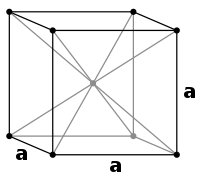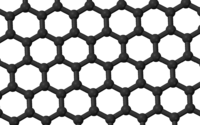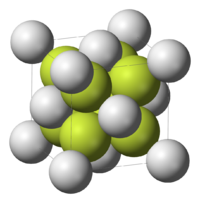# Coordination number

﻿
Coordination number

In chemistry and crystallography, the coordination number of a central atom in a molecule or crystal is the number of its nearest neighbours. This number is determined somewhat differently for molecules and for crystals.

In chemistry, the emphasis is on bonding structure in molecules or ions and the coordination number of an atom is determined by simply counting the other atoms to which it is bonded (by either single or multiple bonds). For example, [Cr(NH3)2Cl2Br2]1- has Cr3+ as its central cation, which has a coordination number of 6.

However the solid-state structures of crystals often have less clearly defined bonds, so a simpler model is used, in which the atoms are represented by touching spheres. In this model the coordination number of an atom is the number of other atoms that it touches. For an atom in the interior of a crystal lattice, the number of atoms touching the given atom is the bulk coordination number; for an atom at a surface of a crystal, this is the surface coordination number.

## Chemistry usage

In chemistry, coordination number (c.n.), defined originally in 1893 by Alfred Werner, is the total number of neighbours of a central atom in a molecule or ion. Although a carbon atom has four chemical bonds in most stable molecules, the coordination number of each carbon is four in methane (CH4), three in ethylene (H2C=CH2, each C is bonded to 2H + 1C = 3 atoms), and two in acetylene. In effect we count the first bond (or sigma bond) to each neighbouring atom, but not the other bonds (pi bonds).

In inorganic chemistry also, only the first or sigma bond between each ligand and the central atom counts, but not any pi bonds to the same ligands. In tungsten hexacarbonyl, W(CO)6, the coordination number of tungsten (W) is counted as six although pi as well as sigma bonding is important in such metal carbonyls.

Examples of high coordination number complexes are the ions formed by uranium and thorium with bidentate nitrate ion ligands, U(NO3)62− and Th(NO3)62−. Here each nitrate ligand is bound to the metal by two oxygen atoms, so that the total coordination number of the U or Th atom is 12.

When the surrounding ligands get smaller even higher coordination numbers may be possible. One computational chemistry study predicted a particularly stable PbHe152+ ion composed of a central lead ion coordinated with no fewer than 15 helium atoms.

## Crystallography usageBCC structure of iron. For greater visibility the atoms are indicated by points rather than touching spheres.

In materials science, the bulk coordination number of a given atom in the interior of a crystal lattice is the number of atoms touching the given atom. Iron at 20oC has a body-centered cubic (BCC) crystal in which each interior iron atom occupies the centre of a cube formed by eight neighbouring iron atoms. The bulk coordination number for this structure is therefore 8.

The highest bulk coordination number is 12, found in both hexagonal close-packed (HCP) and cubic close-packed (CCP) (also known as face-centered cubic or FCC) structures. This value of 12 corresponds to the theoretical limit of the kissing number problem when all spheres are identical.One layer of a graphite crystal (graphene) with carbon atoms and C-C bonds shown in black.

The two most common allotropes of carbon have different coordination numbers. In diamond, each carbon atom is at the centre of a tetrahedron formed by four other carbon atoms, so the coordination number is four, as for methane. Graphite is made of two-dimensional layers in which each carbon is covalently bonded to three other carbons. Atoms in other layers are much further away and are not nearest neighbours, so the coordination number of a carbon atom in graphite is 3 as in ethylene.Calcium fluoride unit cell. In this image the Ca2+(gray) and F-(green) ions are shown as touching spheres

Simple ionic structures are described by two coordination numbers, one for each type of ion. Calcium fluoride (CaF2) is an (8, 4) structure, meaning that each cation Ca2+ is surrounded by eight F anion neighbors, and each anion F by four Ca2+. For sodium chloride (NaCl), the numbers of cations and anions are equal, and both coordination numbers are six so that the structure is (6, 6).

For an atom at a surface of a crystal, the surface coordination number is always less than the bulk coordination number. The surface coordination number is dependent on the Miller indices of the surface. In a body-centered cubic (BCC) crystal, the bulk coordination number is 8, whereas, for the (100) surface, the surface coordination number is 4.

## Usage in quasicrystal, liquid and other disordered systemsFirst coordination number of Lennard-Jones fluidSecond coordination number of Lennard-Jones fluid

The coordination number of systems with disorderness cannot be precisely defined.

The first coordination number can be defined using the radial distribution function g(r)$n_1 = 4 \pi \int_{r_0}^{r_1} r^2 g(r) \rho \, dr,$

where r0 is the rightmost position starting from r = 0 whereon g(r) is approximately zero, r1 is the first minimum. Therefore, it is the area under the first peak of g(r).

The second coordinate number is defined similarly:$n_2 = 4 \pi \int_{r_1}^{r_2} r^2 g(r) \rho \, dr.$

Alternative definitions for the coordination number can be found in literature, but in essence the main idea is the same. One of those definition are as follows: Denote rp as the position of the first peak,$n_1' = 4 \pi \times 2 \int_{r_0}^{r_p} r^2 g(r) \rho \, dr.$

The first coordination shell is the spherical shell with radius between r0 to r1 around the central particle under investigation.

Wikimedia Foundation. 2010.

### Look at other dictionaries:

• coordination number — Crystall. the number of anions surrounding a single cation in a stable crystal structure. [1905 10] * * * ▪ chemistry also called  Ligancy,         the number of atoms, ions, or molecules that a central atom or ion holds as its nearest neighbours …   Universalium

• coordination number — koordinacijos skaičius statusas T sritis Standartizacija ir metrologija apibrėžtis Atomų ar atomų grupių, tiesiogiai prisijungusių prie atomo, skaičius kompleksiniame junginyje. atitikmenys: angl. coordination number vok. Koordinationszahl, f rus …   Penkiakalbis aiškinamasis metrologijos terminų žodynas

• coordination number — koordinacijos skaičius statusas T sritis Standartizacija ir metrologija apibrėžtis Kristalo kurio nors atomo (jono) artimiausių gretimų ir vienodai nutolusių atomų (jonų) skaičius. atitikmenys: angl. coordination number vok. Koordinationszahl, f… …   Penkiakalbis aiškinamasis metrologijos terminų žodynas

• coordination number — koordinacinis skaičius statusas T sritis chemija apibrėžtis Ligandų, supančių kompleksodarį, skaičius. atitikmenys: angl. coordination number; coordination valency rus. координационная валентность; координационное число ryšiai: sinonimas –… …   Chemijos terminų aiškinamasis žodynas

• coordination number — koordinacinis skaičius statusas T sritis chemija apibrėžtis Kristalizacinės gardelės atomą (molekulę) supančių atomų (molekulių) skaičius. atitikmenys: angl. coordination number rus. координационное число …   Chemijos terminų aiškinamasis žodynas

• coordination number — noun 1. : the number of attachments being usually four or six to the central atom in a coordination complex 2. : a number used in classifying various arrangements in space of constituent groups of crystals, the number being a function of the… …   Useful english dictionary

• coordination number — noun Date: 1908 1. the number of attachments to the central atom in a coordination complex 2. a number used in specifying the spatial arrangement of the constituent groups of crystals …   New Collegiate Dictionary

• coordination number — noun the number of ligands surrounding a central metal atom in a coordination compound …   Wiktionary

• coordination number — /koʊˌɔdəneɪʃən ˈnʌmbə/ (say koh.awduhnayshuhn numbuh) noun 1. the number of outer, or ligand, ions bonded to the central atom in a co ordination compound; ligancy. 2. the number of atoms or ions surrounding a central atom or ion in a crystal;… …   Australian English dictionary

• coordination number — the number of atomic or ionic nearest neighbors …   Mechanics glossary